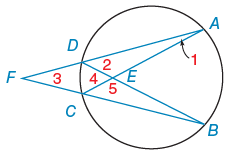Alyssa Z.

# Given: mAB = 94°, mDA = 118°  ,    mBC = 136°. Find the following in degrees.

Consider the following figure.mAB= 94° mDA= 118° mBC= 136°

Find the following in degrees.

(a)m∠1 (∠DAC)

°

°

(c) m∠3 (∠AFB)

°

(d) m∠4 (∠DEC)

°

(e) m∠5 (∠CEB)